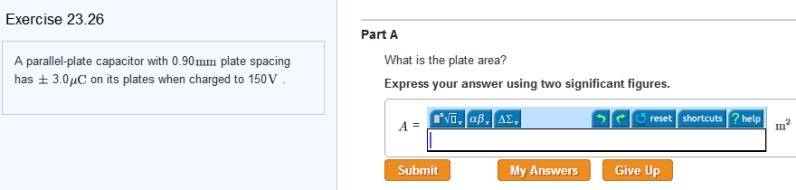# Find the area of the plate

## Homework Statement## Homework Equations

capacitance = charge/volt

capacitance = Esub0 (side of parallel plates^2)/(distance apart)

## The Attempt at a Solution

capacitance = (3*10^-6 C)/(150v)
capacitance = 2 * 10 ^ - 8 F

2 * 10 ^ - 8 F = (8.85 * 10 ^ -12)(side of parallel plate) ^ 2/(.90 *10^-2)

side of parallel plate is 20 m ^ 2.

Last edited:

gneill
Mentor

## Homework Statement## Homework Equations

capacitance = charge/volt

capacitance = Esub0 (side of parallel plates^2)/(distance apart)

## The Attempt at a Solution

capacitance = (3*10^-6 C)/(150v)
capacitance = 2 * 10 ^ - 8 F

2 * 10 ^ - 8 F = (8.85 * 10 ^ -12)(side of parallel plate) ^ 2/(.90 *10^-2)
Your distance value doesn't look right; check your units conversion. Since you're looking for the plate area anyways, why involve the square of the side length when you can solve directly for the area?

Fix your d value and try again. Make sure that your result description and units match (you reported a side length in square meters before).

Your distance value doesn't look right; check your units conversion. Since you're looking for the plate area anyways, why involve the square of the side length when you can solve directly for the area?

Fix your d value and try again. Make sure that your result description and units match (you reported a side length in square meters before).

oops milli is 10^ -3

i found my mistake. plate area = (capacitance)(distance)/(esub0)

plate area = (2*10^-8)(.90 * 10 ^-3)/ (8.85 * 10 ^ -12) = 2 m ^ 2 =]

thanks gneill

gneill
Mentor
oops milli is 10^ -3

i found my mistake. plate area = (capacitance)(distance)/(esub0)

plate area = (2*10^-8)(.90 * 10 ^-3)/ (8.85 * 10 ^ -12) = 2 m ^ 2 =]

Yup, that looks better